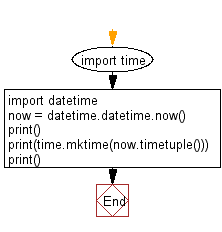﻿ Python: Convert a date to timestamp - w3resource

# Python: Convert a date to timestamp

## Python Datetime: Exercise-30 with Solution

Write a Python program to convert a date to a timestamp.

Sample Solution:

Python Code:

``````import time
import datetime
now = datetime.datetime.now()
print()
print(time.mktime(now.timetuple()))
print()
```
```

Sample Output:

```1494224813.0
```

Flowchart:Python Code Editor: